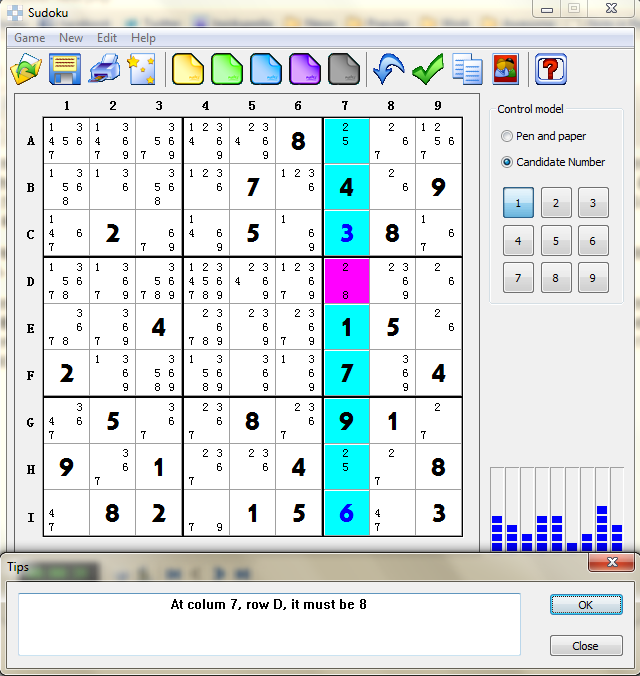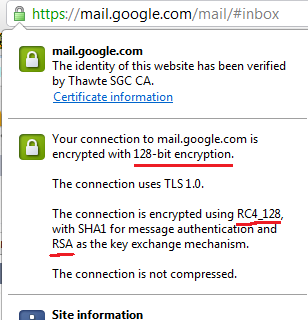当年做的蛮开心的一个小程序浅谈公钥密码前篇文章谈到一个非对称密钥加密系统，觉得很是神奇，一直想找一个实例，最近查阅了一些资料，google了一下，找到一些，分享一下。

(1) 选取两个大素数p和q (保密)

(2) 计算n=pq（公开的系统参数），γ=(p-1)(q-1)(保密)

(3) 随机选取整数e（公开的加密密钥），使得gcd(e, γ)=1

(4) 计算d（保密,私人密钥），使得ed≡1(mod γ),即d=e-1(mod γ)

(5) 加密:c=me mod n

(6) 解密:m=cd mod n

p = 11, q = 23，则 n = pq = 253

γ=(p-1)(q-1) = 220